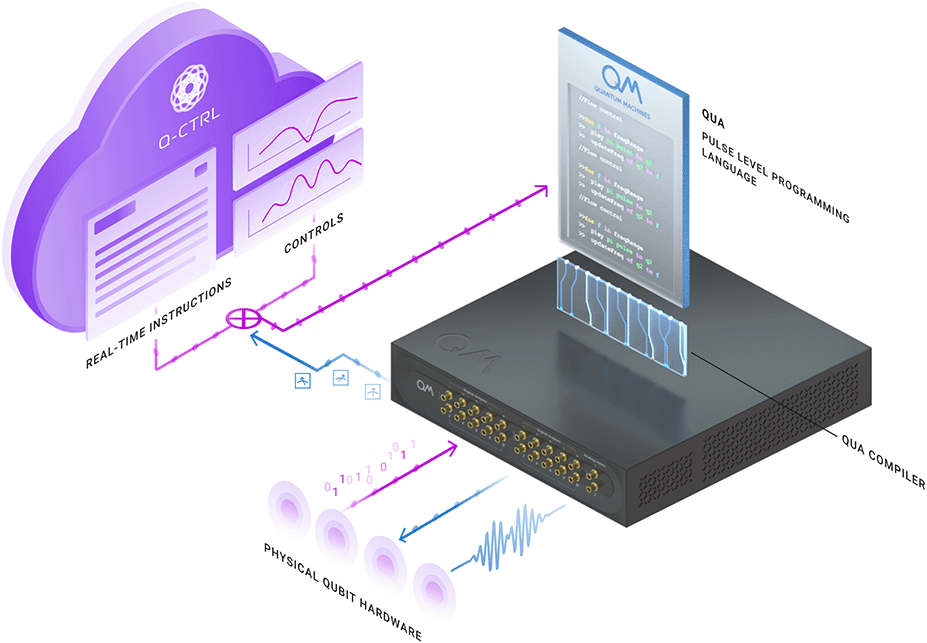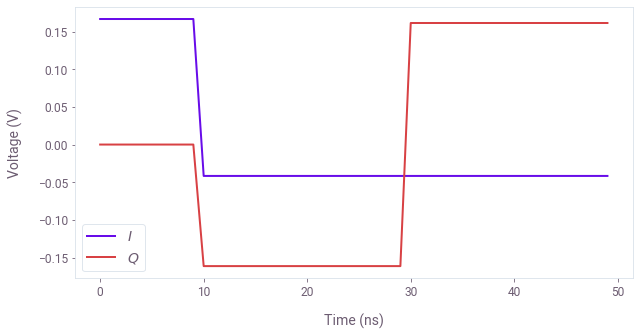# How to integrate Boulder Opal with QUA from Quantum Machines

Integrate Boulder Opal pulses directly into Quantum Machines hardware using the Q-CTRL QUA Python package

Boulder Opal enables you to design and benchmark noise-robust pulses, as well as characterize and tune up your quantum hardware with ease. This process becomes even simpler and more powerful when software and hardware are seamlessly integrated.

In this notebook we show how to interface Boulder Opal with QUA, a programming language developed by Quantum Machines that provides pulse-level control of their Operator-X (OPX) quantum devices. Using the qctrl-qua package, we show how to convert a pulse into an OPX-compatible format and to quickly generate the configurations required for executing experiments.Some cells in this notebook require access to Quantum Machines OPX Arbitrary Waveform Generator controlling a superconducting qubit system to execute properly. Your individual system specifics may vary from what is shown here.

## Summary workflow

### 1. Define the QUA configuration

Before you can run any experiment, you first need to provide QUA with a thorough description of the quantum device. This “QUA configuration” is a Python dictionary containing instructions for configuring the QM hardware. It typically contains line calibrations, drive frequencies and pulse shapes for quantum gates. For more details, see the qua examples here.

### 2. Convert Q-CTRL pulses to QUA-compatible pulses

Now that you have a pre-existing QUA configuration, qm_config, you can take the Q-CTRL optimized pulse and add it to your QUA pulse library. For convenience, we will use add_pulse_to_config function from the qctrlqua package. This function accepts qm_config and a piecewise-constant (PWC) pulse sampled at 1ns intervals and returns an appropriately-formatted Python dictionary that you can feed into the OPX. For more details, see the qctrlqua documentation here.

## Worked example:

In the following, we will import a pulse from the Q-CTRL Open Controls library, described in the reference documentation and convert it into a QUA-compatible format.

import numpy as np
import matplotlib.pyplot as plt

# Predefined pulse imports
import qctrlopencontrols

# Q-CTRL imports

from qctrlvisualizer import get_qctrl_style

plt.style.use(get_qctrl_style())


Here we show the example of an SK1 $\pi$-pulse but the exact shape of the pulse we import is not important to illustrate the conversion. The pulse is resampled to match the 1ns resolution of the hardware.

total_rotation = np.pi  # Target rotation angle here is a \pi pulse.
omega_max = 2 * np.pi * 50e6  # Hz, this is the maximum Rabi rate in the system.

control_pulse = qctrlopencontrols.new_sk1_control(
rabi_rotation=total_rotation, azimuthal_angle=0.0, maximum_rabi_rate=omega_max
)

dt = 1e-9  # hardware time resolution
resampled = control_pulse.resample(dt)


The next step is to map the units of the optimal pulse (rad/s) onto the values of the input amplitudes of the hardware. As an illustration, here we plot the $I$ and $Q$ values obtained using a typical experimental value for rabi_rate. In general, this value would have to be determined by running a standard Rabi experiment in your setup.

rabi_rate = 2 * np.pi * 300e6
qctrl_i, qctrl_q = resampled.amplitude_x / rabi_rate, resampled.amplitude_y / rabi_rate

plt.figure(figsize=(10, 5))
plt.plot(qctrl_i, label="$I$")
plt.plot(qctrl_q, label="$Q$")
plt.xlabel("Time (ns)")
plt.ylabel("Voltage (V)")
plt.legend()
plt.show()To add the pulse to the QUA configuration you just need to input it into the add_pulse_to_config function. Note that you also need to specify the hardware channel that’s going to be used.

qm_config = {}
# Add the Q-CTRL robust pulse to the QUA configuration
pulse_name = "Q-CTRL"
channel_name = "drive_channel"  # corresponds to the drive channel name in qm_config
qm_config = add_pulse_to_config(pulse_name, channel_name, qctrl_i, qctrl_q, qm_config)


You are now ready to use the updated qm_config dictionary and run the QUA program on the hardware.